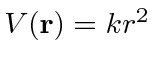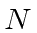## Sample Test Problems

1. A particle of massin 3 dimensions is in a potential. Find the energy eigenstates in terms of 3 quantum numbers. What is the energy of the ground state and first excited state?
2. * N identical fermions are bound (at low temperature) in a potential. Use separation in Cartesian coordinates to find the energy eigenvalues in terms of a set of three quantum numbers (which correspond to 3 mutually commuting operators). Find the Fermi energy of the system. If you are having trouble finding the number of states with energy less than, you may assume that it is.
3. A particle of mass m is in the potential. Find operators that commute with the Hamiltonian and use them to simplify the Schrödinger equation. Solve this problem in the simplest way possible to find the eigen-energies in terms of a set of "quantum numbers" that describe the system.
4. A particle is in a cubic box. That is, the potential is zero inside a cube of side L and infinite outside the cube. Find the 3 lowest allowed energies. Find the number of states (level of degeneracy) at each of these 3 energies.
5. A particle of mass m is bound in the 3 dimensional potential.
a)
Find the energy levels for this particle.
b)
Determine the number of degenerate states for the first three energy levels.
6. A particle of massis in a cubic box. That is, the potential is zero inside a cube of sideand infinite outside.
a)
Find the three lowest allowed energies.
b)
Find the number of states (level of degeneracy) at each of these three energies.
c)
Find the Fermi Energyforparticles in the box. (N is large.)
7. A particle is confined in a rectangular box of length, width, and tallness''. Find the energy eigenvalues in terms of a set of three quantum numbers (which correspond to 3 mutually commuting operators). What are the energies of the three lowest energy states if,, and.
8. A particle of mass m is bound in the 3 dimensional potential.
9. a) Find the energy levels for this particle.
10. b) Determine the number of degenerate states for the first three energy levels.
11. In 3 dimensions, a particle of massis bound in a potential.
a)
The definite energy states will, of course, be eigenfunctions of. What other operators can they be eigenfunctions of?
b)
Simplify the three dimensional Schrdinger equation by using these operators.

Jim Branson 2013-04-22